Question

# Determine the internal normal force, shear force, and moment at point D.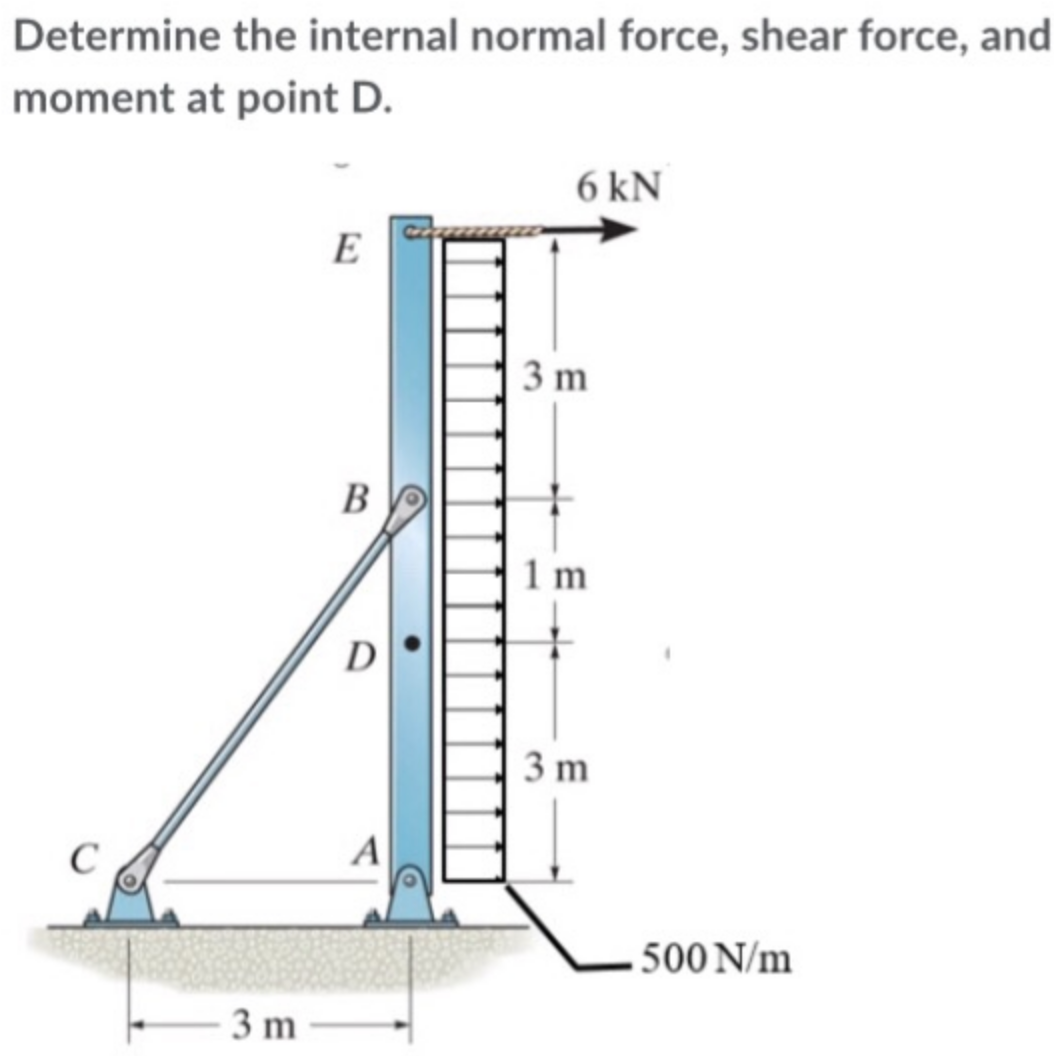Determine the internal normal force, shear force, and moment at point D.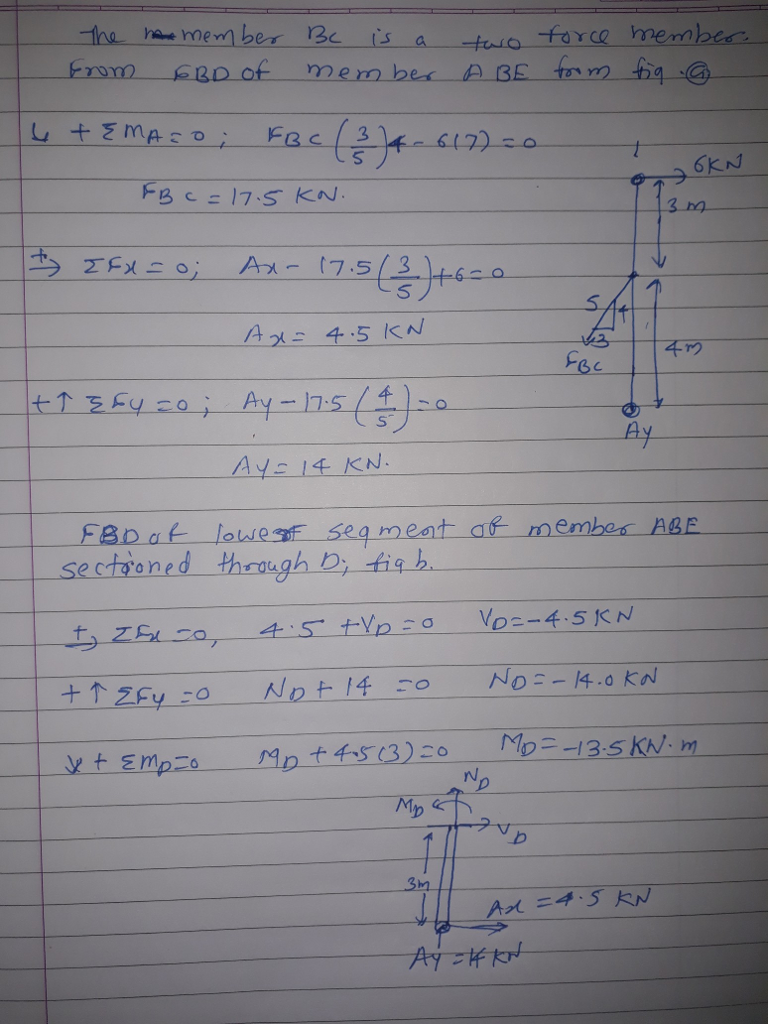#### Earn Coins

Coins can be redeemed for fabulous gifts.

Similar Homework Help Questions
• ### Determine the internal normal force, internal shear force, and internal moment at points D and E of the frame.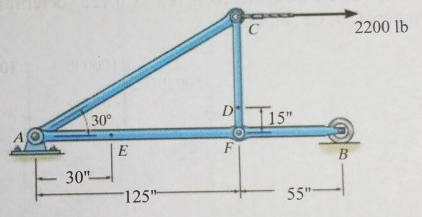Determine the internal normal force, internal shear force, and internal moment at points D and E of the frame.

• ### Determine the internal normal force, shear force, and moment at points C and D in the...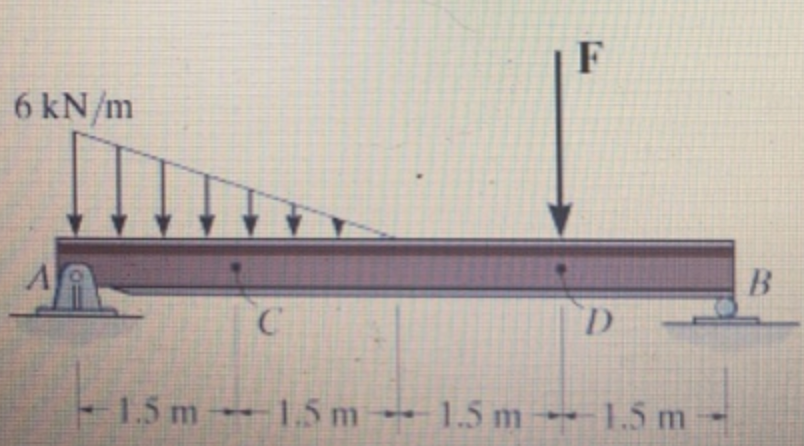Determine the internal normal force, shear force, and moment at points C and D in the simply supported beam. Point D is located just to the left of the F = 15 kN concentrated load. (Figure 1)

• ### Determine the internal normal force, shear force, and the moment at points C and D.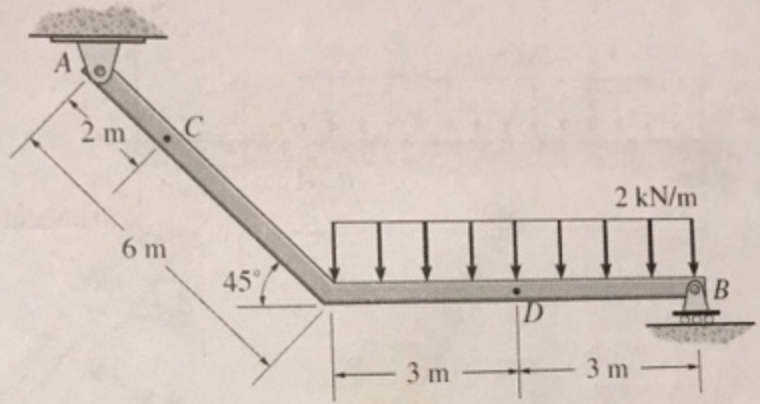Determine the internal normal force, shear force, and the moment at points C and D.

• ### Determine the internal normal force, shear force, and bending moment at point C in the beam.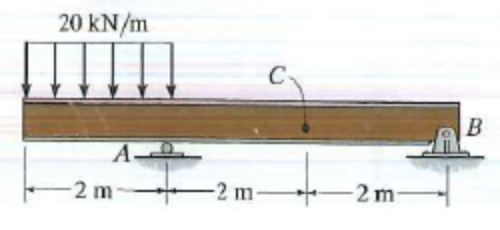Determine the internal normal force, shear force, and bending moment at point C in the beam.

• ### Determine the internal normal force, shear force, and moment at points and D in the simply supported beam.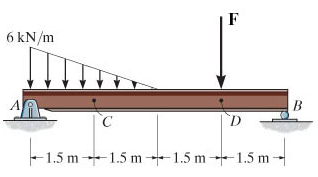Determine the internal normal force, shear force, and moment at points and D in the simply supported beam. Point Dis located just to the left of the F = 14 kN concentrated load.(Figure 1)

• ### Determine the internal normal force, shear force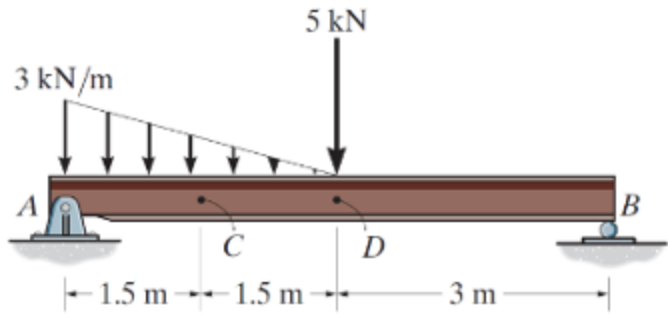Determine the internal normal force, shear force, and moment at points C and D in the simply supported beam using method of sections. Point D is A located just to the left of the 5-kN force. Also, draw shear and moment diagrams using the graphical method.

• ### Determine the normal force, shear force, and moment at point C.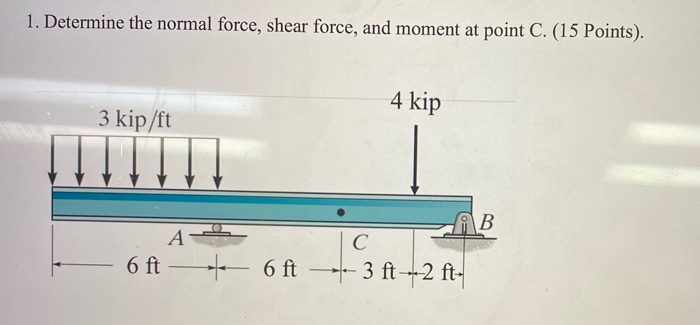Determine the normal force, shear force, and moment at point C.

• ### Determine the normal force, shear force, and moment at point C.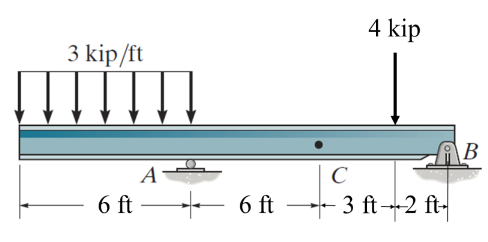Determine the normal force, shear force, and moment at point C.

• ### If w0 = 320 N/m, determine the internal normal force, shear force, and moment at point E of the two-member frame. (Figure 1)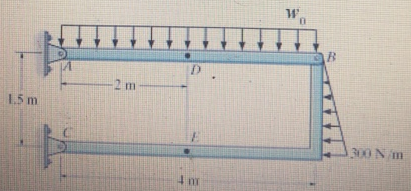If w0 = 320 N/m, determine the internal normal force, shear force, and moment at point E of the two-member frame. (Figure 1)

• ### Calculate the internal normal force, internal shear force, and internal bending moment at a location 0.75m...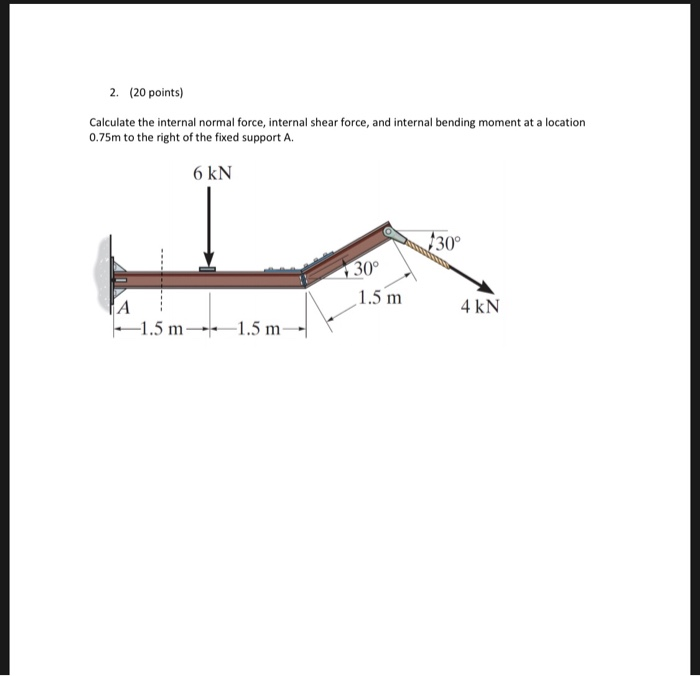Calculate the internal normal force, internal shear force, and internal bending moment at a location 0.75m to the right of the fixed support A. 2. (20 points) Calculate the internal normal force, internal shear force, and internal bending moment at a location 0.75m to the right of the fixed support A 6 kN 30° 30° 1.5 m 4 kN 1.5 m1.5 m